Exceptional subvariety

A closed subvarietyof an algebraic variety, defined over an algebraically closed field, that can be mapped onto a subvarietyof lesser dimension by a proper birational morphism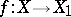such that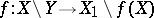is an isomorphism (cf. also Proper morphism). The morphismis called a contraction ofonto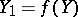; this concept is a particular case of that of a modification of algebraic spaces . If,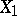,,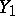are smooth irreducible varieties,is called an exceptional subvariety of the first kind. Ifhas codimension 1 in, it is an exceptional divisor. Exceptional divisors on an algebraic surface are called exceptional curves.

The notion of an exceptional subvariety can be naturally generalized to schemes, algebraic and complex-analytic spaces. The corresponding morphisms are called contractions; the notion of an exceptional subvariety of the first kind can also be naturally generalized. An exceptional subvariety in a complex-analytic space is also called an exceptional analytic set.

Characterizing the exceptional subvarieties within the ambient variety is one of the basic problems in birational geometry. Historically, the first example of such a characterization is the Enriques–Castelnuovo criterion: An irreducible complete curveon a smooth surfaceis an exceptional subvariety of the first kind if and only if it is isomorphic to the projective lineand if its self-intersection index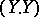onis equal to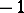(cf. , ). This criterion can be generalized to one-dimensional subschemes of a two-dimensional regular scheme (cf. , ). Ifis an arbitrary connected complete curve with irreducible components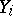on a smooth projective surface, then a necessary (but not sufficient) condition forto be exceptional is that the matrix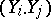is negative definite (cf. ). In the case of a connected complex curve on a smooth complex surface, or a connected complete curve on a smooth two-dimensional algebraic space, the analogous condition is necessary and sufficient for exceptionality.

The multi-dimensional analogue of the Enriques–Castelnuovo criterion for contractions to a point has the following form : An irreducible complete subvarietyin a smooth algebraic varietyis exceptional of the first kind relative to a contraction to a point if the following two conditions hold: a), where; and b) the normal bundle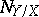toinis defined by a divisor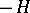, whereis a hyperplane in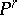. In this caseis projective. The corresponding contractionis a monoidal transformation with centre at the point(cf. , ).

In the analytic case, necessary and sufficient conditions for a connected compact complex submanifoldin a complex manifoldto be exceptional of the first kind have been found; the corresponding contractionmust be a monoidal transformation with centre at(cf. Exceptional analytic set). For algebraic varieties the corresponding conditions are necessary, but not always sufficient.

For a contractionof an exceptional subvariety of the first kindin a projective algebraic varietyonto a zero-dimensional subvarietyin an algebraic variety,need not be projective. Moreover, if the algebraic varietiesandare defined over the field of complex numbers, under an analytic contractionof the exceptional subvariety of the first kind, the varietyneed not be algebraic at any point, in general.

Regarding the question of contractibility of an exceptional subvariety (not necessarily of the first kind) to a point, a necessary condition of exceptionality of a complete connected algebraic subspacein a smooth algebraic spaceis that the normal bundleis negative (this condition is not sufficient for). The analogous fact holds for complex spaces.

In the case of algebraic spaces, the most general criterion of exceptionality states that in the category of Noetherian algebraic spaces a subspaceinis an exceptional subvariety if and only if the formal completionin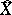is an exceptional subvariety in the category of formal algebraic spaces . In other words, contraction of algebraic subspaces is possible if and only if it is possible for the corresponding formal completions.